Previous: Moving an Object Up or Down the Stacking Order  Up: Selecting and Editing Objects  Next: Scaling Objects

# Rotating Objects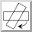Selected objects can be rotated either by clicking on the rotate button or by selecting Transform->Rotate (Ctrl-W). This will display a dialog box in which you can specify the angle of rotation.

Notes:

• Individual objects will be rotated relative to the centre of the object.

• Objects within a group will be rotated relative to the centre of the group.

• Rotating a text-path will rotate the path and the text will adjust to follow the transformed path.

• Rotating a text area and path and then combining them to form a text-path is not the same as first combining and then rotating.

To illustrate this, in Figure 8.17(a) there are three objects selected. The selection is then rotated 90°. The result is shown in Figure 8.17(b).

In Figure 8.18, the three objects in Figure 8.17 were first grouped (Figure 8.18(a)) and then rotated 90° (Figure 8.18(b)).

In Figure 8.19, the path and text area in Figure 8.19(a) are combined into a text-path, shown in Figure 8.19(b). This text-path is then rotated by 90° resulting in Figure 8.19(c). Note that this is different from first rotating the original path and text area, shown in Figure 8.19(d), and then combining them to form a text-path, shown in Figure 8.19(e).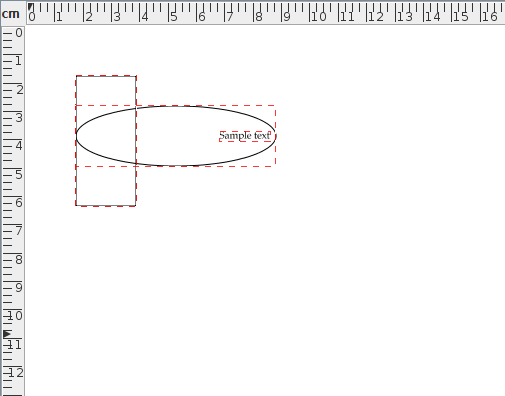(a)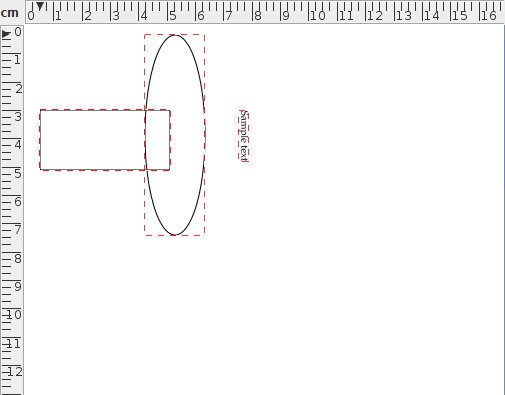(b)
Figure 8.17: Three selected objects rotated by 90 degrees: (a) before, (b) after.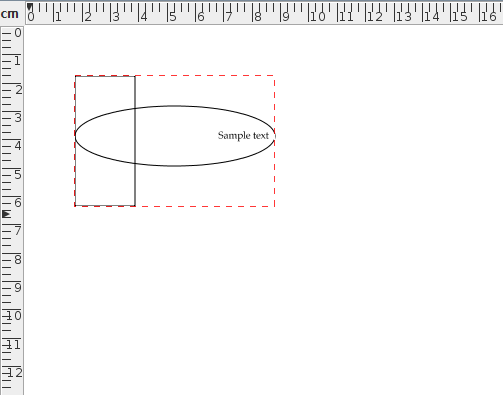(a)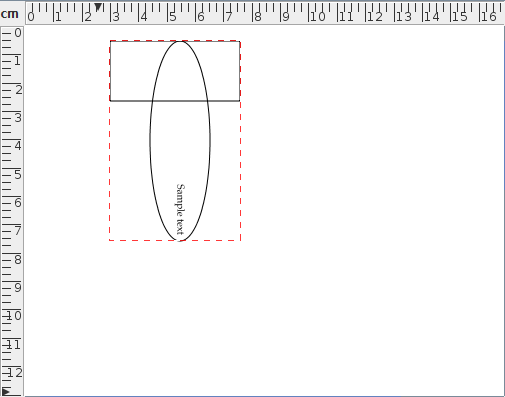(b)
Figure 8.18: A group consisting of three objects rotated by 90 degrees: (a) before, (b) after.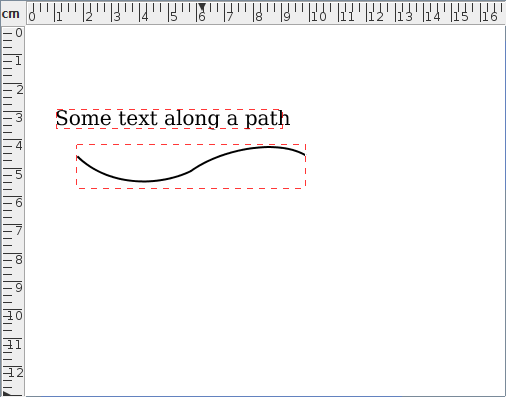(a)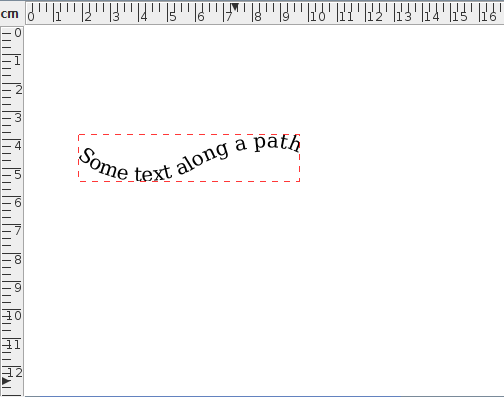(b)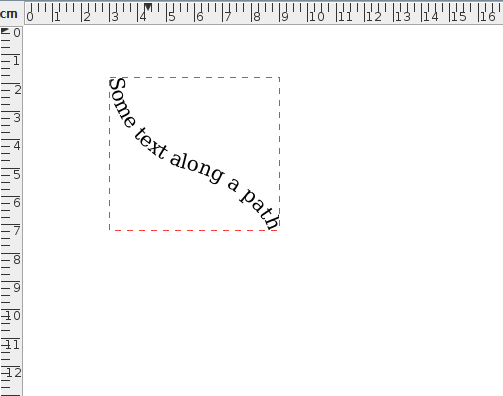(c)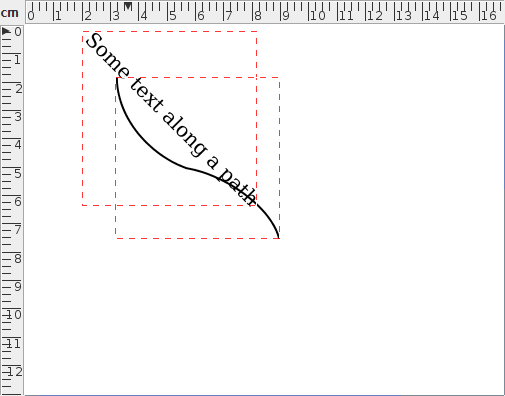(d)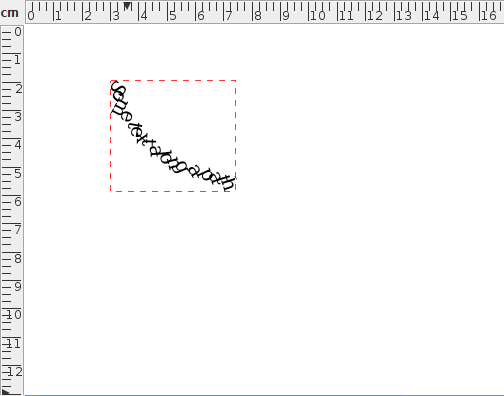(e)
Figure 8.19: Rotating a text-path: (a) original text area and path; (b) text area and path in (a) combined to form a text-path; (c) text-path in (b) rotated by 45 degrees; (d) text area and path in (a) rotated by 45 degrees; (e) rotated text area and path in (d) combined to form a text-path.

If you prefer to rotate an object using the mouse, you first need to enable the hotspots. Then drag the bottom left hotspot to rotate. Note that even if you have more than one object selected, only the object whose hotspot you are dragging will be transformed.